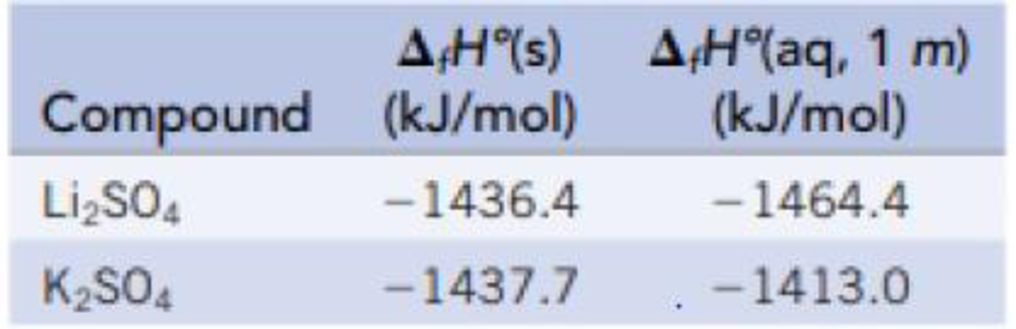Chapter 13, Problem 73GQ

Chapter
Section
Textbook Problem

Calculate the enthalpies of solution for Li2SO4 and K2SO4. Are the solution processes exothermic or endothermic? Compare them with LiCl and KCl. What similarities or differences do you find?Interpretation Introduction

Interpretation: The enthalpy of solution of Li2SO4 and K2SO4 is to be identified and to find out whether the solution process is endothermic or exothermic. The enthalpy of solution of Li2SO4 and is to be compared with the enthalpy of solution of LiCl and KCl

Concept introduction:

Enthalpy of solution: In the process, the change in enthalpy while dissolving the solute in the solvent.

The enthalpy of solution is calculated by the formula

ΔsolnH0=Σ(nΔfH0(product))Σ(nΔfH0(reactant))

Explanation

Given,

Enthalpy of formation of Li2SO4in the solid state =1436.4 kJ/molEnthalpy of formation of Li2SO4in the solution    = 1464.4 kJ/molEnthalpy of formation of K2SO4in the solid state =1437.7 kJ/molEnthalpy of formation of K2SO4in the solution    = 1413.0 kJ/mol

The enthalpy of solution is calculated by the formula

ΔsolnH0=Σ(nΔfH0(product))Σ(nΔfH0(reactant))

The enthalpy of solution of Li2SO4 is,

ΔsolnH0=ΔfHo[Li2SO4(aq)]-ΔfHo[Li2SO4(s)](wheren=1forboth)=(1464.4 kJ/mol)(1436.4 kJ/mol)=28 kJ/mol

The enthalpy of solution of K2SO4 is 24.7 kJ/mol

The value of ΔsolnH0 is negative and hence it is an exothermic process

The enthalpy of solution of K2SO4 is,

ΔsolnH0=ΔfHo[K2SO4(aq)]-ΔfHo[K2SO4(s)](wheren=1forboth)=(1413

Still sussing out bartleby?

Check out a sample textbook solution.

See a sample solution

The Solution to Your Study Problems

Bartleby provides explanations to thousands of textbook problems written by our experts, many with advanced degrees!

Get Started

Find more solutions based on key concepts